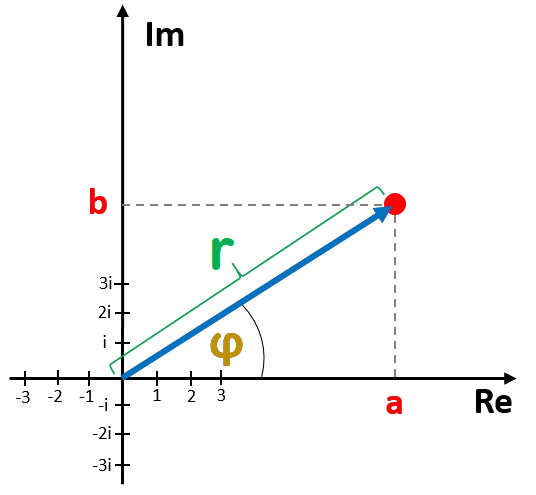# Complex Number to Trigonometric Form Calculator

Created by Anna Szczepanek, PhD
Reviewed by Komal Rafay
Last updated: May 04, 2023

This complex number to trigonometric form calculator will help if you're still not quite sure how to find the trigonometric form of a complex number a + bi. In the brief article below, we explain what the trig form of a complex number is and how to quickly convert from rectangular to trigonometric form.

## What is the trigonometric form of a complex number?

The trigonometric form of a complex number z reads

z = r × [cos(φ) + i × sin(φ)],

where:

• r is the modulus, i.e., the distance from (0,0) to z; and
• φ is the argument, i.e., the angle between the x-axis and the radius between (0,0) and z.

You can see r and φ in the picture below. Using basic trigonometry, we see that
cos(φ) = a × r and sin(φ) = b × r, exactly as claimed by the formula above.## How do I find the trigonometric form of a complex number?

To convert a complex number z = a + bi from rectangular to trigonometric form, you need to determine both the magnitude and the argument of z:

1. Compute the magnitude as r = √(a² + b²).
2. Compute the phase as φ = atan(b / a). If needed, correct by ±π to end up in the correct quadrant of the plane.
3. Write down the trigonometric form as z = r × [cos(φ) + i × sin(φ)].

## How to use this complex number to trigonometric form calculator

To use the power of our tool, you need to feed it with the real and imaginary parts of your complex number, so with a and b from the a + bi form.

Our complex number to trigonometric form calculator will immediately compute the magnitude r and phase (argument) φ of your number and then display the trigonometric form in two ways: using radians and degrees.

🙋 For some particular complex numbers you will get a third form as well: it will use π × rad as the angle unit. In this way, angles like π/4 have a chance of appearing.

## Omni calculators and complex numbers

Satisfied with the complex number to trigonometric form calculator? Omni Calculator features a rich collection of calculators dedicated to complex numbers! Here's a full list in case you want to learn more:

## FAQ

### What is the trigonometric form of i+1?

The answer is i = √2 × [cos(π/4) + i × sin(π/4)]. To arrive at this result, observe that the magnitude of i equals r = √(1² + 1²) = √2. Then observe that the argument of i equals π/4 since atan(1/1) = π/4.

### How do I get the trigonometric form from polar form?

If you have a complex number in the polar form r × exp(iφ), it suffices to take r and φ from the polar form and write down r × [cos(φ) + i × sin(φ)] to get the trig form of your complex number. No calculations required!

Anna Szczepanek, PhD
Rectangular form
Real part (a)
Imaginary part (b)
Trigonometric form
Magnitude |z₁|
Phase (φ₁)
deg
People also viewed…

### Circumference

Use this free circumference calculator to find the area, circumference and diameter of a circle.

### Midpoint

Easily find the midpoint between two Cartesian coordinates using our midpoint calculator. Master the midpoint formula or calculate instantly!

### Plastic footprint

Find out how much plastic you use throughout the year with this plastic footprint calculator. Rethink your habits, reduce your plastic waste, and make your life a little greener.

### Right trapezoid area

If you're looking to calculate the area of a right trapezoid, this is the right tool for you!